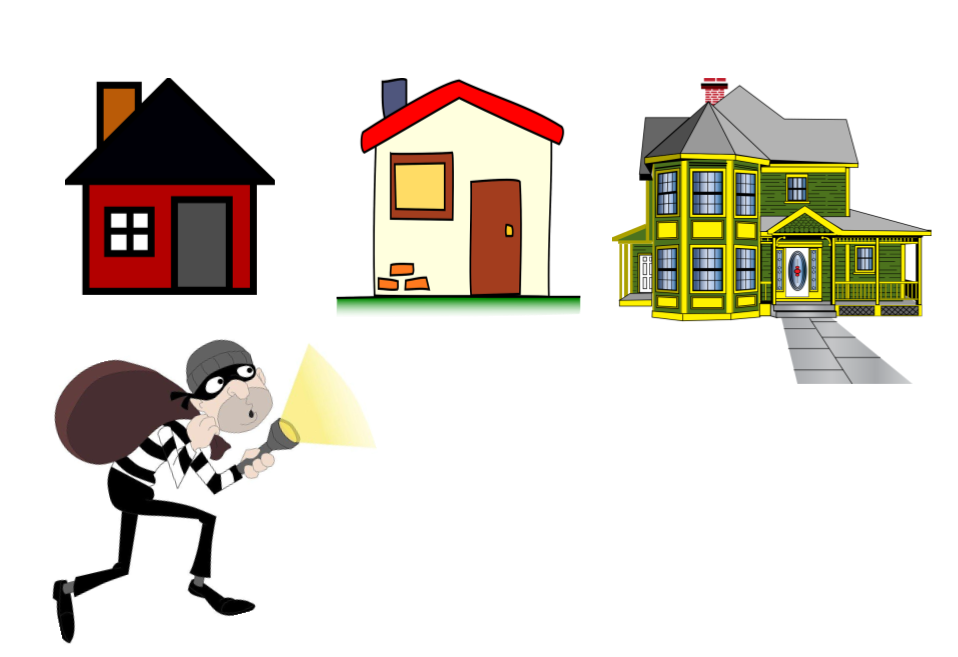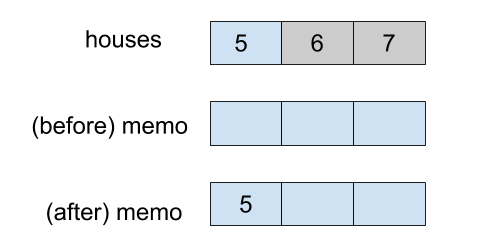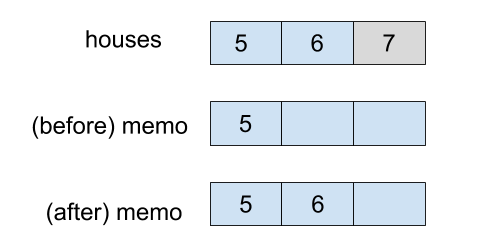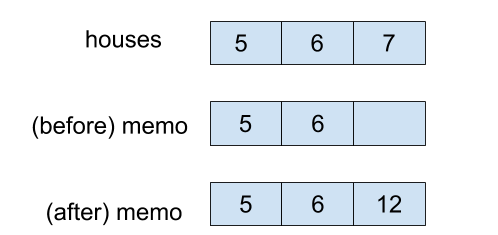×

Search anything:

# Maximum houses a Robber can rob

#### Algorithms Dynamic Programming (DP)There are several houses in a society where the security system of two adjacent house is connected. Hence, if a robber robs two adjacent house, police is informed automatically and the robber does not want this to happen. The robber has the information of how much money is stored in each house. The robber needs to rob alternative houses to be safe.

The robber wants to know how many houses can be robbed without informing the police.

Given a list of non-negative integers representing the amount of money of each house, determine the maximum amount of money the robber can rob tonight without alerting the police. We have solved this problem using Dynamic Programming.## Intuition

If the robber robs two adjacent houses, alarm will set off. Thus, the robber should rob alternate houses.

e.g [5,6,7]
Consider each element in array represents the amount of money each house has.
The robber has following choices of houses to rob
choice a: 5 (0-th house) and 7 (2-nd house)
choice b: 6 (1-st house)

Obviously, the robber will go with the choice a, as 5 + 7, gives 12 which is the maximum amount he can rob. Now that we have solved the problem, we need to write code for the same. This is where dynamic programming comes into picture.

# Solution using Dynamic Programming (DP)

Now, house robber is a famous problem which is to be solved by dynamic programming. What if you don't know that? Well, I did not know that. After trying really hard to solve it myself, I finally gave up and reached out to get some help. Let me try to save some efforts for you by presenting a series of questions you have to ask yourselves to solve any dynamic programming problem.

1. Is this a dynamic programming problem?
2. What's the base case?
3. What is the recurrence relation?
5. Should be solved iteratively or recursively?
6. What is the time and space complexity?

# Is this a dynamic programming problem?

If you can think of the problem solution as a function of solutions to smaller subproblems, it is a clue that it is a candidate for recursion and hence optimization using dynamic programming. As dynamic programming is nothing but an optimization technique over recursion.

e.g Factorial (3) = 3 * Factorial(2) = 3 * Factorial(2) * Factorial(1)
If we can solve Factorial(2) and Factorial(1), we have solution to original problem Factorial (3).

Let's try to break our house robber problem into smaller subproblems.

# What's the base case?

We will first consider the smallest possible subproblem of the given problem and try to solve it. We always tend to know the answer to this smallest possible subproblem.

Say the street has only one house e.g  The robber will only rob that house as it has no other choice. This smallest possible subproblem will form the base case for the recursion.

We could then increase the problem size one by one and solve these subsequent subproblems. Also, let's try and find if there is any relation in between these subproblems.

# What is the recurrence relation?

Consider a street with two houses e.g. [5, 6]
The robber will rob the house with maximum amount of money i.e. 6 (1-st index) in this case.

How about a street with three houses? e.g [5, 6, 7]
When robber is at 1st house (0-th index), the robber is clueless if he should rob it or leave it. As next house might have larger stash. So he is going sneak in the 1st house, keep a note of its stash and move ahead.

Following similar approach he will sneak into the last house.
Now, the robber has two choices:

1. Rob the current house and the house before its immediate neighbor i.e. 7 (2-th index) and 5 (0-th index), total amount he can rob becomes 12.
2. Rob maximum of immediate neighbor and the house before it i.e. Maximum(5, 6). The maximum amount he can rob with this choice is 6.

So which of the choices the robber choose? Remember, robber wants to get maximum amount. So he will rob maximum of these two choices.

Let's try to write this down in the form of a relation.
Considering that robber is at currently at the last house, say n is the last house on this street.

1. rob(n) + rob(n-2)
2. Maximum(rob(n-2), rob(n-1))
Result of robbery = Maximum(choice 1, choice 2)

Memoization is nothing but calculating solution of each subproblem only once and storing it for future use.

Now if a street has more than three houses, the robber will sneak into the house, consider it as a last one and make a note of the amount he will get by following above decision making process. In real world, robber can use a notebook to make a note or memo and in programming world we can use an array. Say we are using an array named as memo. How will our memo look like as our robber progresses through all the houses one by one:

1. 1st house visited:2. 1st and 2nd houses visited:(before) memo = [5, ?], ? = maximum [5, 6] = 6
(after) memo = [5, 6]
3. 1st, 2nd, 3rd houses visited:(before) memo = [5, 6, ?]
? = Maximum[rob(n) + rob(n-2), Maximum(rob(n-1), rob(n-2)]
? = Maximum[rob(n) + memo(n-2), memo(n-1)]
Notice, how we are leveraging already stored results from the memo.
? = Maximum[7 + 5, 6) = 12
(after) memo = [5, 6, 12]

# Should be solved iteratively or recursively?

Iterative Solution:

``````class Solution {
public int rob(int[] nums) {
if (nums == null || nums.length == 0) {
return 0;
} else if (nums.length == 1) {
return nums;
}
int[] memo = new int[nums.length];
memo = nums;
memo = Math.max(nums, nums);
for (int i = 2; i < nums.length; i++) {
memo[i] = Math.max(memo[i - 2] + nums[i],
memo[i - 1]);
}
return memo[nums.length - 1];
}
}
``````

Recursive Solution:

``````class Solution {
public int rob(int[] nums) {
if (nums == null || nums.length == 0) {
return 0;
}
int memo[] = new int[nums.length];
// we fill -1 since a house can have 0 money.
Arrays.fill(memo, -1);
return rob(nums, memo, nums.length - 1);
}

private int rob(int[] nums, int[] memo, int n) {
// this can happen due to our recursive calls
if (n < 0) {
return 0;
} else if (memo[n] >= 0) {
return memo[n];
}
memo[n] = Math.max(rob(nums, memo, n - 2) + nums[n], rob(nums, memo, n - 1));
return memo[n];
}
}
``````

I prefer iterative solutions because

• No stack overflow fear.
• We can take full advantage of memoization.

# What is the time and space complexity?

Time complexity: O(N), n is number of elements in the input array
Space complexity: O(N), space is required for the memo array

With this article at OpenGenus, you must have the complete idea of solving this problem efficiently using Dynamic Programming. Enjoy.#### Priya Raut

An enterprise software developer proficient in Java | A believer 'to teach is to learn twice' | A newbie writer striving for excellence

Maximum houses a Robber can rob